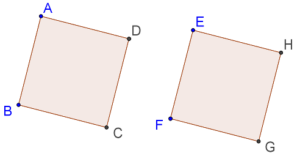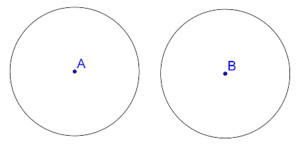# What is Congruence?

What is Congruence?

## What is Congruence?

Two geometrical figures are said to be congruent if they are identical in every respects.

For example, two squares of the same side-length are congruent, as shown below:Similarly, two circles with the same radius are congruent:If two geometrical figures are congruent, they can be exactly superimposed upon each other.

Note: If a figure $$A$$ is congruent to a figure $$B$$, we will write this fact as follows: $$A \cong B$$\. Notice the following obvious facts about congruence:

1. If $$A \cong B$$, then $$B \cong A$$. This simply means that saying that $$A$$ is congruent to $$B$$ is the same as saying that $$B$$ is congruent to $$A$$.

2. If $$A \cong B$$ and $$B \cong C$$, then $$A \cong C$$. This should once again be easy to understand. If $$A$$ is congruent to $$B$$, it can be superimposed exactly on $$B$$. Similarly, $$B$$ can be superimposed exactly on $$C$$. Thus, $$A$$ can be superimposed exactly on $$C$$, and hence $$A \cong C$$.

grade 9 | Questions Set 1
grade 9 | Questions Set 2
More Important Topics
Numbers
Algebra
Geometry
Measurement
Money
Data
Trigonometry
Calculus
More Important Topics
Numbers
Algebra
Geometry
Measurement
Money
Data
Trigonometry
Calculus